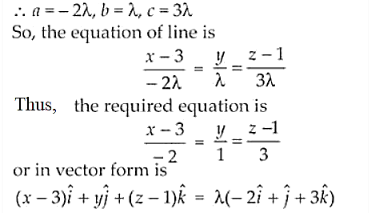# Find the equations of the line passing through the point (3,0,1)

Question:

Find the equations of the line passing through the point (3,0,1) and parallel to the planes + 2= 0 and 3– = 0.

Solution:

Given point is (3, 0, 1) and the equation of planes are

x + 2y = 0 …. (i) and 3y – z = 0 …. (ii)

Equation of any line l passing through (3, 0, 1) is

l: (x – 3)/a = (y – 0)/b = (z – 1)/c

Now, the direction ratios of the normal to plane (i) and plane (ii) are (1, 2, 0) and (0, 3, 1).

As the line is parallel to both the planes, we have

1.a + 2.b + 0.c = 0 ⇒ a + 2b + 0c = 0 and

0.a + 3.b – 1.c = 0 ⇒ 0a + 3b – c = 0

So,          $\frac{a}{-2-0}=\frac{-b}{-1-0}=\frac{c}{3-0}=\lambda$